Name:    3rd Grade Basic Math Test 7

Multiple Choice
Identify the choice that best completes the statement or answers the question.

1.

Number Sense and Numeration – Decimals – RIT 181 – 190

Which decimal is less than 14.26?
 a. 14.62 d. 14.60 b. 41.22 e. 15.06 c. 14.2

2.

Number Sense and Numeration – Fractions – RIT 181 – 190Which picture shows an equivalent amount?
 a.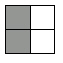d.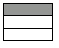b.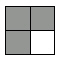e.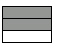c.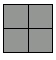3.

Reduce 16/20 to lowest terms.
 a. 8/10 d. 4/5 b. 20/16 e. 2/3 c. 3/4

4.

In what place is the letter o in the word skateboard?
 a. third d. tenth b. ninth e. seventh c. fifth

5.

Which comparison is correct?
 a. 946 > 964 d. 3,126 < 2,316 b. 6,462 > 6,642 e. 398 > 389 c. 861 < 618

6.

Which is in order from least to greatest?
 a. 8/10, 7/10, 5/10 d. 4/5, 3/10, 1/2 b. 3/8, 1/2, 3/4 e. 1/2, 1/3, 1/6 c. 1/6, 1/8, 1/3

7.

Which set is in order from least to greatest?
 a. 2.36, 2.45, 2.19 d. 10.40, 9.63, 11.05 b. 8.42, 8.98, 9.14 e. 1.98, 1.89, 1.80 c. 3.68, 3.65, 3.46

8.

Number Sense and Numeration – Place Value – RIT 181 – 190
How would you write the number 82,460 in word form?
 a. eighty-two thousand, four hundred six b. eighty-two thousand, six hundred forty c. eight hundred two thousand, four hundred sixty d. eight thousand, two hundred forty-six e. eighty-two thousand, four hundred sixty

9.

In which place is the digit 4 in the number 146,253?
 a. thousands d. ten thousands b. millions e. hundreds c. hundred thousands

10.

3 hundreds 4 tens 6 ones + 3 tens + 2 ones
 a. 379 d. 369 b. 378 e. 648 c. 666

11.

In which place is the digit 3 in 78.536?
 a. tens d. ones b. hundredths e. tenths c. thousandths

12.

Number Sense and Numeration – Whole Numbers – RIT 181 – 190

What number is one less than 328?
 a. 319 d. 327 b. 326 e. 329 c. 328

13.

Which set counts by 3’s?
 a. 12, 15, 18, 21 d. 15, 18, 22, 27 b. 13, 15, 17, 19 e. 6, 9, 11, 16 c. 3, 9, 15, 21

14.

Complete the number pattern.
1,410, 1,415, 1,420, ____, ____
 a. 1,425, 1,430 d. 425, 430 b. 1,421, 1,422 e. 1,520, 1,620 c. 1,430, 1,440

15.

Round to the nearest hundred.
862
 a. 800 d. 862 b. 860 e. 960 c. 900

16.

Roman Numerals I, V, and X have the value of _________.
 a. 10, 15, 20 d. 1, 5, 10 b. 5, 10, 15 e. 1, 25, 50 c. 1, 10, 20

17.

Which number can be divided by 5 with no remainder?
 a. 7,319 d. 1,042 b. 2,980 e. 6,543 c. 5,287

18.

What is the greatest common factor of 54 and 72?
 a. 9 d. 1 b. 2 e. 12 c. 3

19.

What are the two common multiples of 4 and 6?
 a. 18, 20 d. 16, 28 b. 30, 40 e. 12, 24 c. 8, 10

20.

Problem Solving – RIT 161 – 170

There are 5 blue bears and 4 red bears. How many bears are there in all?
 a. 6 d. 7 b. 10 e. 1 c. 9